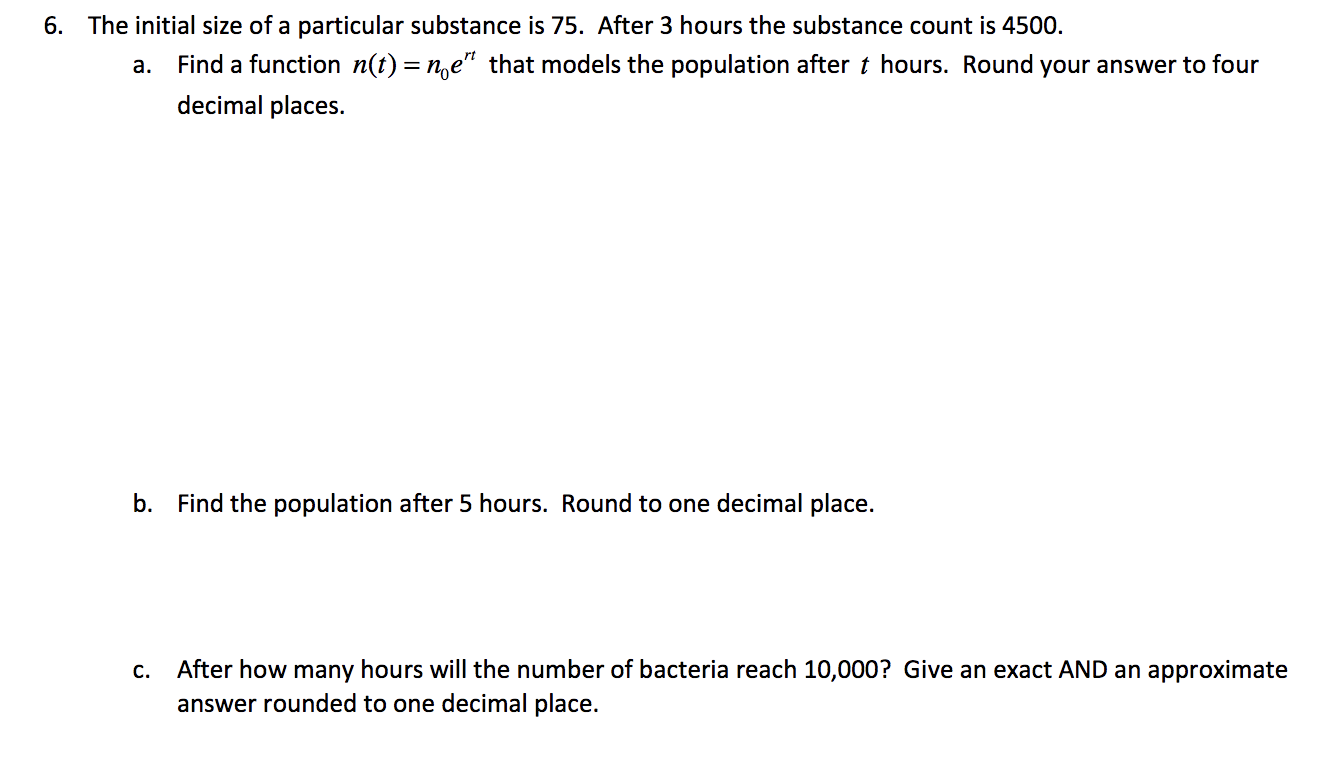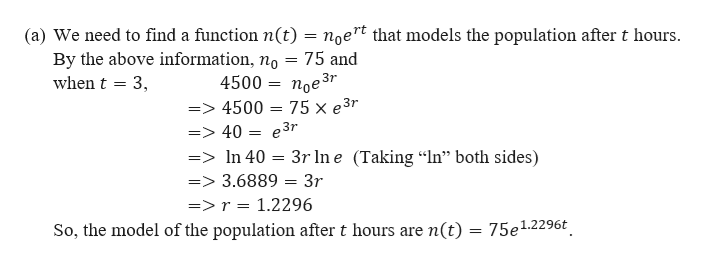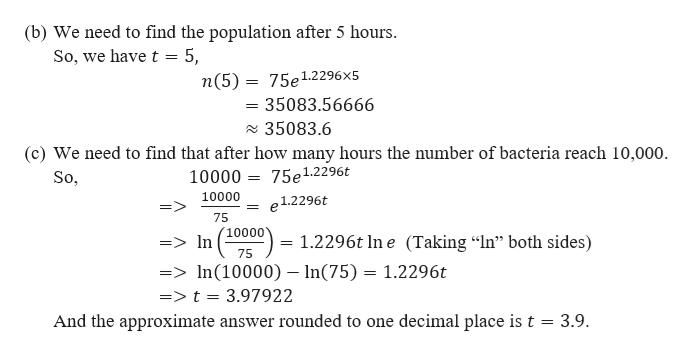# The initial size of a particular substance is 75. After 3 hours the substance count is 4500.6.Find a function n(t) = n,e" that models the population after t hours. Round your answer to fourа.decimal places.b.Find the population after 5 hours. Round to one decimal place.After how many hours will the number of bacteria reach 10,000? Give an exact AND an approximateanswer rounded to one decimal place.С.

Question
1 viewshelp_outlineImage TranscriptioncloseThe initial size of a particular substance is 75. After 3 hours the substance count is 4500. 6. Find a function n(t) = n,e" that models the population after t hours. Round your answer to four а. decimal places. b. Find the population after 5 hours. Round to one decimal place. After how many hours will the number of bacteria reach 10,000? Give an exact AND an approximate answer rounded to one decimal place. С. fullscreen
check_circle

Step 1

We are given that the initial size of a particular substance is 75 and after 3 hours the substance count is 4500.help_outlineImage Transcriptionclose(a) We need to find a function n(t) = noert that models the population after t hours By the above information, no = 75 and when t 3 4500 = noe3r => 4500 = 75 x e3r => 40 = e3r =>In 40 3r Ine Taking "In" both sides) => 3.6889 = 3r =>r = 1.2296 So, the model of the population after t hours are n(t) = 75e1.2296t fullscreen
Step 2

Now,

...help_outlineImage Transcriptionclose(b) We need to find the population after 5 hours So, we have t 5, n(5) 75e1.2296 x5 =35083.56666 35083.6 (c) We need to find that after how many hours the number of bacteria reach 10,000. 10000 75e1.2296t So, 10000 e1.2296t 75 10000 In 1.2296t In e (Taking "In" both sides) => = 75 =>In(10000) - In(75) 1.2296t =>t = 3.97922 And the approximate answer rounded to one decimal place is t = 3.9. fullscreen

### Want to see the full answer?

See Solution

#### Want to see this answer and more?

Solutions are written by subject experts who are available 24/7. Questions are typically answered within 1 hour.*

See Solution
*Response times may vary by subject and question.
Tagged in

### Functions